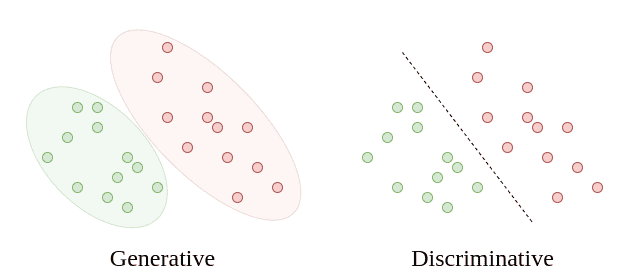## 1. Introduction

In this tutorial, we’ll learn about generative and discriminative machine learning algorithms.

First of all, we’ll learn the definitions of generative and discriminative algorithms. Then, we’ll compare and contrast the algorithms to understand their strengths and weaknesses.

## 2. Generative Algorithms

Algorithms of this type try to model “how to populate the dataset.” Sampling the model gives generated, synthetic data points.

We estimate the probability distributions. Formally, the generative model estimates the conditional probabilityfor a given target. For example, the Naive Bayes algorithm modelsand then transforms the probabilities into conditional probabilitiesby applying the Bayes rule.

Some popular generative algorithms are:

• Naive Bayes Classifier
• Gaussian Mixture Model
• Hidden Markov Model
• Probabilistic context-free grammar

## 3. Discriminative Algorithms

Discriminative algorithms focus on modeling a direct solution. For example, the logistic regression algorithm models a decision boundary. Then it decides on the outcome of an observation based on where it stands relative to the decision boundary.

Discriminative algorithms estimate posterior probabilities. Unlike the generative algorithms, they don’t model the underlying probability distributions. Formally, we model the conditional probability of targetgiven an observation.

Some popular discriminative algorithms are:

• k-nearest neighbors (k-NN)
• Logistic regression
• Support Vector Machines (SVMs)
• Decision Trees
• Random Forest
• Artificial Neural Networks (ANNs)

## 4. Generative vs. Discriminative Models

Let’s assume our task is to determine the language of a text document. How do we solve this task with the help of machine learning?

We can learn each language and then determine the language. This is how generative models work.

Alternatively, we can learn just the linguistic differences and common patterns of languages without actually learning the language. This is the discriminative approach. In this case, we don’t speak any language.

In other words, discriminative algorithms focus on how to distinguish cases. Hence, they focus on learning a decision boundary. On the other hand, generative algorithms learn the fundamental properties of the data and how to generate it from scratch:The generative approach focuses on modeling, whereas the discriminative approach focuses on a solution. So, we can use generative algorithms to generate new data points. Discriminative algorithms don’t serve that purpose.

Still, discriminative algorithms generally perform better for classification tasks. That’s because they focus on solving the actual problem directly instead of solving a more general problem first.

Yet, the real strength of generative algorithms lies in their ability to express complex relationships between variables. In other words, they have explanatory power. As a result, they have successful use cases in NLP and medicine.

On the other hand, discriminative algorithms feel like black-boxes, without the ability to express their decision boundaries in simple terms. The relationships between variables are not explicitly explainable. Therefore, we can’t visualize it easily.

Besides, generative models are suited to solve unsupervised learning tasks, as well as supervised learning tasks, since they have predictive ability. Discriminative models require labeled datasets and can’t deduce from a context. Consequently, generative models have more comprehensive applications in anomaly detection and monitoring areas.

Moreover, generative algorithms converge faster than discriminative algorithms. Thus, we prefer generative models when we have a small training dataset.

Even though the generative models converge faster, they converge to a higher asymptotic error. On the contrary, the discriminative models converge to a smaller asymptotic error. So, as the number of training examples increases, the error rate decreases for the discriminative models.

To summarize, generative and discriminative algorithms have their own strengths and weaknesses: